# pathmetrics

Information for path metrics

Since R2019b

## Description

The `pathmetrics` object holds information for computing path metrics. Use object functions to calculate smoothness, clearance, and path validity based on a set of poses and the associated map environment.

## Creation

### Syntax

``pathMetricsObj = pathmetrics(path)``
``pathMetricsObj = pathmetrics(path,validator)``

### Description

````pathMetricsObj = pathmetrics(path)` creates an object based on the input `navPath` object. The state validator is assumed to be a `validatorOccupancyMap` object, if the state space of the `navPath` object is a `stateSpaceSE2`, `stateSpaceDubins`, or `stateSpaceReedsShepp` object. Otherwise, The state validator is assumed to be a `validatorOccupancyMap3D` object, if the state space of the `navPath` object is a `stateSpaceSE3` object. The `path` input sets the value of the Path property.```

example

````pathMetricsObj = pathmetrics(path,validator)` creates an object based on the input `navPath` object and associated state validator for checking the path validity. The `validator` input sets the value of the StateValidator property.```

## Properties

expand all

Path data structure, specified as a `navPath` object is the path whose metric is to be calculated.

Validator for states on path, specified either as a `validatorOccupancyMap`, `validatorVehicleCostmap`, or `validatorOccupancyMap3D` object.

For 2-D state spaces, The default state validator is `validatorOccupancyMap(stateSpaceSE2,binaryOccupancyMap(10))`.

For 3-D state spaces, The default state validator is `validatorOccupancyMap3D(stateSpaceSE3)`.

## Object Functions

 `clearance` Minimum clearance of path `isPathValid` Determine if planned path is obstacle free `show` Visualize path metrics in map environment `smoothness` Smoothness of path

## Examples

collapse all

Compute smoothness, clearance, and validity of a planned path based on a set of poses and the associated map environment.

Load and Assign Map to State Validator

Create an occupancy map from an example map and set the map resolution.

```load exampleMaps.mat; % simpleMap mapResolution = 1; % cells/meter map = occupancyMap(simpleMap,mapResolution);```

Create a Dubins state space.

`statespace = stateSpaceDubins;`

Create a state validator based on occupancy map to store the parameters and states in the Dubins state space.

`statevalidator = validatorOccupancyMap(statespace);`

Assign the map to the validator.

`statevalidator.Map = map;`

Set the validation distance for the validator.

`statevalidator.ValidationDistance = 0.01;`

Update the state space bounds to be the same as the map limits.

`statespace.StateBounds = [map.XWorldLimits;map.YWorldLimits;[-pi pi]];`

Plan Path

Create an RRT* path planner and allow further optimization.

```planner = plannerRRTStar(statespace,statevalidator); planner.ContinueAfterGoalReached = true;```

Reduce the maximum number of iterations and increase the maximum connection distance.

```planner.MaxIterations = 2500; planner.MaxConnectionDistance = 0.3;```

Define start and goal states for the path planner as `[`x, y, theta`]` vectors. x and y are the Cartesian coordinates, and theta is the orientation angle.

```start = [2.5, 2.5, 0]; % [meters, meters, radians] goal = [22.5, 8.75, 0];```

Plan a path from the start state to the goal state. The plan function returns a `navPath` object.

```rng(100,'twister') % repeatable result [path,solutionInfo] = plan(planner,start,goal);```

Compute and Visualize Path Metrics

Create a path metrics object.

`pathMetricsObj = pathmetrics(path,statevalidator);`

Check path validity. The result is `1 (true)` if the planned path is obstacle free. `0 (false)` indicates an invalid path.

`isPathValid(pathMetricsObj)`
```ans = logical 1 ```

Calculate the minimum clearance of the path.

`clearance(pathMetricsObj)`
```ans = 1.4142 ```

Evaluate the smoothness of the path. Values close to `0` indicate a smoother path. Straight-line paths return a value of `0`.

`smoothness(pathMetricsObj)`
```ans = 1.7318 ```

Visualize the minimum clearance of the path.

```show(pathMetricsObj) legend('Planned Path','Minimum Clearance')```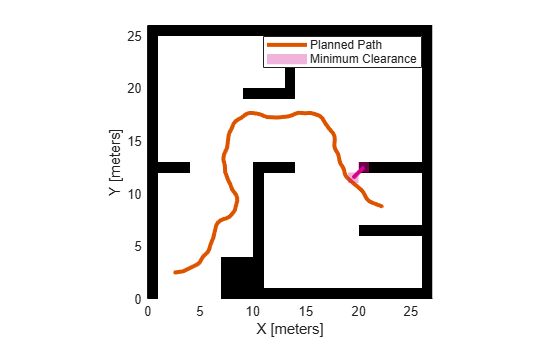Plan a vehicle path through a parking lot using the RRT* algorithm. Compute and visualize the smoothness, clearance, and validity of the planned path.

Load and Assign Map to State Validator

Load a costmap of a parking lot. Plot the costmap to see the parking lot and the inflated areas that the vehicle should avoid.

```load parkingLotCostmap.mat; costmap = parkingLotCostmap; plot(costmap) xlabel('X (meters)') ylabel('Y (meters)')```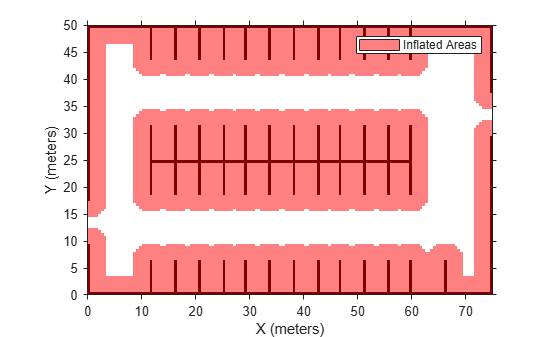Create a `stateSpaceDubins` object and increase the minimum turing radius to `4` meters.

```statespace = stateSpaceDubins; statespace.MinTurningRadius = 4; % meters```

Create a `validatorVehicleCostmap` object using the created state space.

`statevalidator = validatorVehicleCostmap(statespace);`

Assign the parking lot costmap to the state validator object.

`statevalidator.Map = costmap;`

Plan Path

Define start and goal poses for the vehicle as [`x`, `y`, `Θ`] vectors. World units for the (`x`,`y`) locations are in meters. World units for the orientation angles `Θ` are in degrees.

```startPose = [5, 5, 90]; % [meters, meters, degrees] goalPose = [40, 38, 180]; % [meters, meters, degrees]```

Use a `pathPlannerRRT` (Automated Driving Toolbox) object and the `plan` (Automated Driving Toolbox) function to plan the vehicle path from the start pose to the goal pose.

```planner = pathPlannerRRT(costmap); refPath = plan(planner,startPose,goalPose);```

Interpolate along the path at every one meter. Convert the orientation angles from degrees to radians.

```poses = zeros(size(refPath.PathSegments,2)+1,3); poses(1,:) = refPath.StartPose; for i = 1:size(refPath.PathSegments,2) poses(i+1,:) = refPath.PathSegments(i).GoalPose; end poses(:,3) = deg2rad(poses(:,3));```

Create a `navPath` object using the Dubins state space object and the states specified by `poses`.

`path = navPath(statespace,poses);`

Compute and Visualize Path Metrics

Create a `pathmetrics` object.

`pathMetricsObj = pathmetrics(path,statevalidator);`

Check path validity. The result is `1` (`true`) if the planned path is obstacle free. `0` (`false`) indicates an invalid path.

`isPathValid(pathMetricsObj)`
```ans = logical 1 ```

Compute and visualize the minimum `clearance` of the path.

`clearance(pathMetricsObj)`
```ans = 0.5000 ```
```show(pathMetricsObj) legend('Inflated Areas','Planned Path','Minimum Clearance') xlabel('X (meters)') ylabel('Y (meters)')```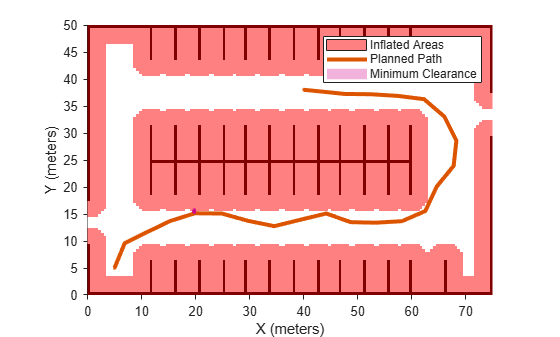Compute and visualize the `smoothness` of the path. Values close to `0` indicate a smoother path. Straight-line paths return a value of `0`.

`smoothness(pathMetricsObj)`
```ans = 0.0842 ```
```show(pathMetricsObj,'Metrics',{'Smoothness'}) legend('Inflated Areas','Path Smoothness') xlabel('X (meters)') ylabel('Y (meters)')```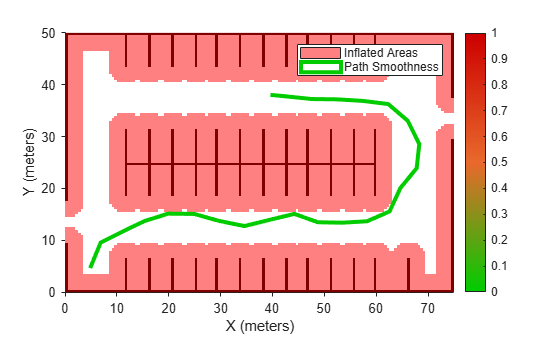Visualize the clearance for each state of the path.

```show(pathMetricsObj,'Metrics',{'StatesClearance'}) legend('Inflated Areas','Planned Path','Clearance of Path States') xlabel('X (meters)') ylabel('Y (meters)')```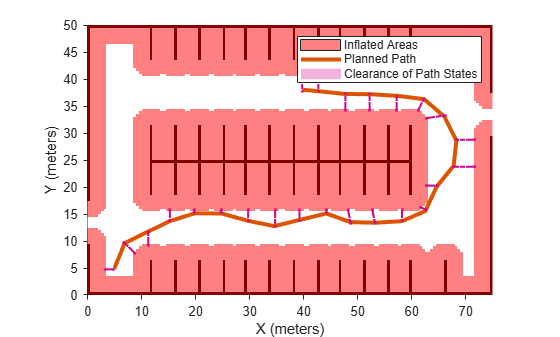Plan a path through a city block using the RRT algorithm. Compute and visualize the smoothness, clearance, and validity of the planned path.

Load and Assign Map to State Validator

Load a 3-D occupancy map of a city block into the workspace. Specify the threshold to consider cells as obstacle-free.

```mapData = load("dMapCityBlock.mat"); omap = mapData.omap; omap.FreeThreshold = 0.5;```

Inflate the occupancy map to add a buffer zone for safe operation around the obstacles.

`inflate(omap,1)`

Create an SE(3) state space object with bounds for state variables.

`ss = stateSpaceSE3([0 220;0 220;0 100;inf inf;inf inf;inf inf;inf inf]);`

Create a 3-D occupancy map state validator using the created state space. Assign the occupancy map to the state validator object. Specify the sampling distance interval.

```sv = validatorOccupancyMap3D(ss, ... Map = omap, ... ValidationDistance = 0.1);```

Plan Path

Create a RRT path planner with increased maximum connection distance and reduced maximum number of iterations. Specify a custom goal function that determines that a path reaches the goal if the Euclidean distance to the target is below a threshold of 1 meter.

```planner = plannerRRT(ss,sv, ... MaxConnectionDistance = 50, ... MaxIterations = 1000, ... GoalReachedFcn = @(~,s,g)(norm(s(1:3)-g(1:3))<1), ... GoalBias = 0.1);```

Specify start and goal poses.

```start = [50 180 25 0.7 0.2 0 0.1]; goal = [150 33 35 0.3 0 0.1 0.6];```

Configure the random number generator for repeatable result.

`rng(1,"twister")`

Plan the path.

`[pthObj,solnInfo] = plan(planner,start,goal);`

Compute and Visualize Path Metrics

Create a path metrics object.

`pathMetricsObj = pathmetrics(pthObj,sv);`

Check path validity. The result is `1 (true)` if the planned path is obstacle free. `0 (false)` indicates an invalid path.

`isPathValid(pathMetricsObj)`
```ans = logical 1 ```

Calculate the minimum clearance of the path.

`clearance(pathMetricsObj)`
```ans = 10 ```

Evaluate the smoothness of the path. Values close to `0` indicate a smoother path. Straight-line paths return a value of `0`.

`smoothness(pathMetricsObj)`
```ans = 0.0011 ```

Visualize the minimum clearance of the path.

```show(pathMetricsObj) axis equal view([100 75]) hold on % Start state scatter3(start(1,1),start(1,2),start(1,3),"g","filled") % Goal state scatter3(goal(1,1),goal(1,2),goal(1,3),"r","filled") % Path plot3(pthObj.States(:,1),pthObj.States(:,2),pthObj.States(:,3), ... "r-",LineWidth=2)```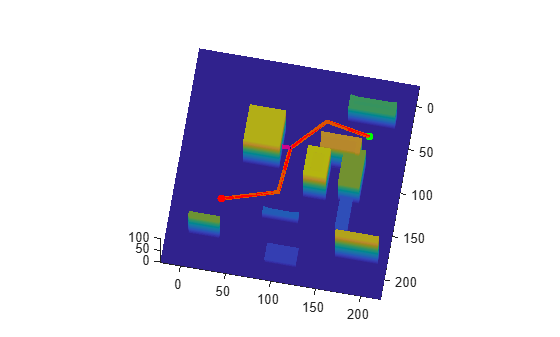## Version History

Introduced in R2019b